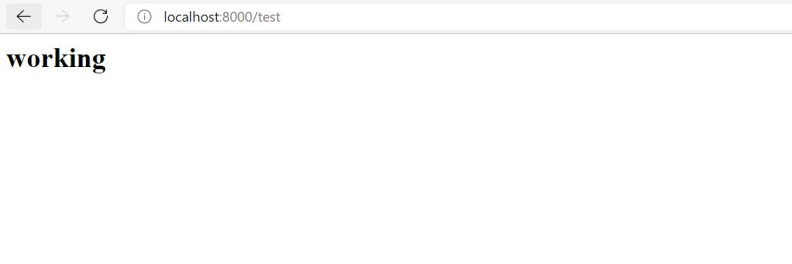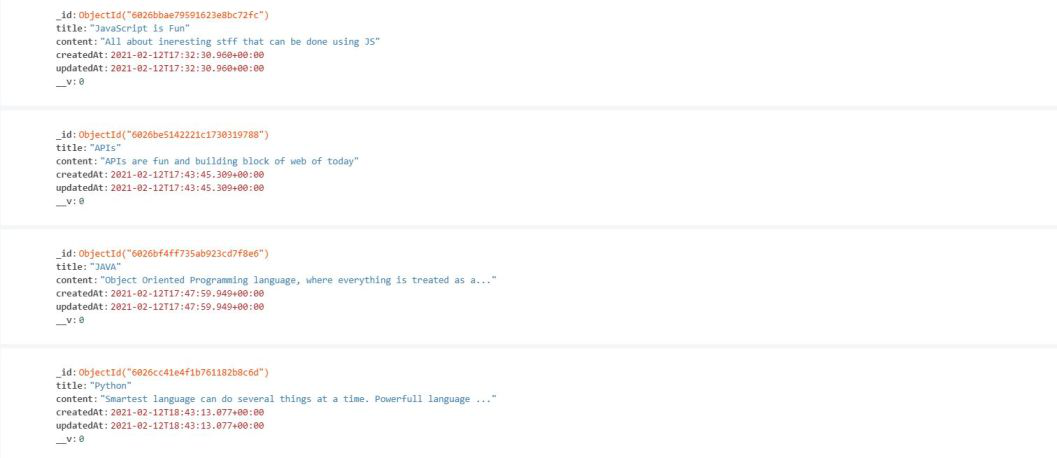Open in App
Not now

# How to limit the number of documents in a MongoDB request in Node.js ?

• Last Updated : 20 Oct, 2021

Say you are working on a quiz API, with which anybody on the web can fetch random questions based on the defined category. You are done with your API and it has passed all test parameters, now for sake of users you want to add a feature that will let users decide how many questions they want to address and your API will provide them with that amount of questions. Here, comes the helpful mongo feature limit.

Referring to mongo documentation, the limit can be understood as an option that limits the number of documents that has to be passed to the next pipeline for processing.

Syntax:

`{ \$limit: <positive integer> }`

For Node.js we will use mongoose for this specific purpose. Assuming that you created a Post model for a Content Management System, let’s try fetching a certain number of posts using the find() method.

## Javascript

 `Post.find().limit(5).then(posts=>{ ` `    ``if``(!posts){ ` `        ``const error=``new` `Error(``"No post is there to fetch"``); ` `        ``error.statusCode=404; ` `        ``throw``(error); ` `    ``} ` `    ``return` `res.status(200).json({post:posts}); ` `}).``catch``(err=>console.log(err));`

Pass the number of documents you want to fetch as an argument to limit. That’s a quite abstraction right? Worry not let’s walk through the process from the very beginning.

Assuming a decent foundation of Node JS, express and mongoose. Starting off with creating posts and fetching posts as per defined limit.

Workflow for follow along will be:

1. Setting up environment
2. Creating Post schema with mongoose
3. Developing API for post creation
4. Developing API for fetching the specified number of post from database

Setting up:

Boiler Plate:

`touch app.js`

Setting up the NPM setup:

`npm init`

Installing dependencies:

`npm i express body-parser mongoose`

Project structure: Now, your file tree will look somewhat.Follow the below steps one by one to achieve the target:

Step 1: Setting up the app.js.

Filename: app.js

## Javascript

 `const express = require(``"express"``);  ` `const bodyParser = require(``"body-parser"``);  ` `const mongoose = require(``"mongoose"``);  ` `   `  `const app = express();  ` `   `  `// For processing application/json  ` `app.use(bodyParser.json());  ` `   `  `// Setting up CORS  ` `app.use((req, res, next) => {  ` `  ``res.setHeader(``"Access-Control-Allow-Origin"``, ``"*"``);  ` `  ``res.setHeader(``"Access-Control-Allow-Methods"``, ``"POST,PUT"``);  ` `  ``res.setHeader(``"Access-Control-Allow-Headers"``, ``"*"``);  ` `  ``next();  ` `});  ` `   `  `app.get(``"/test"``, (req, res, next) => {  ` `  ``res.send(``"

working

"``);  ` `});  ` `   `  `mongoose  ` `  ``.connect(``"mongodb://localhost:27017/articleDB"``)  ` `  ``.then(app.listen(8000, () => console.log(``"listening at port 8000"``)));`

Run the app.js file

`node .\app.js  `

Output: Now on hitting localhost:8000/test, you should expect.Now, when we are done with our basic app setting, lets create our required APIs.

Creating Post Schema: Schemas can be easily created in Node using mongoose.

## Javascript

 `// Requiring mongoose ` `const mongoose = require(``"mongoose"``); ` ` `  `// Schema of our post model ` `const postSchema = ``new` `mongoose.Schema( ` `  ``{ ` `    ``title: { ` `      ``type: String, ` `      ``required: ``true``, ` `    ``}, ` `    ``content: { ` `      ``type: String, ` `      ``required: ``true``, ` `    ``}, ` `  ``}, ` `  ``{ timestamps: ``true` `} ` `); ` ` `  `// Model ` `const Post = mongoose.model(``"Post"``, postSchema);`

API for post creation: Now, as we are done it creating the model and schemas we will move forward creating our first API and then testing it using Postman.

## Javascript

 `// POST http://localhost:8000/post ` `app.post(``"/post"``,(req,res,next)=>{ ` `    ``const newPost = ``new` `Post({ ` `    ``title: req.body.title, ` `    ``content: req.body.content, ` `  ``}); ` `  ``newPost ` `    ``.save() ` `    ``.then((post) => { ` `      ``return` `res.status(201).json({ post: post }); ` `    ``}) ` `    ``.``catch``((err) => { ` `      ``res.status(500).json({error:err}); ` `    ``}); ` `})`

```URL - http://localhost:8000/post
Method - POST
Content-Type - application/json
Body of the request should contain -
i. title - title of your article
ii. content - content of your article```

Output:

API for fetching post as per the queried number of posts: Idea is to pass the count as a query in the API endpoint and then processing that query using basic validation and limit.

## Javascript

 `// GET http://localhost:8000/posts ` ` `  `app.get(``"/posts"``,(req,res,next)=>{ ` `  ``const requestCount = req.query.count; ` `  ``Post.find() ` `    ``.countDocuments() ` `    ``.then((count) => { ` `      ``// if requested count is more than actual count of posts in database ` `      ``if` `(requestCount > count) { ` `        ``const error = ``new` `Error(``"invalid request in quantity"``); ` `        ``error.statusCode = 400; ` `        ``throw` `error; ` `      ``} ` `      ``//returning posts while limiting the count ` `      ``return` `Post.find().limit(Number(requestCount)); ` `    ``}) ` `    ``.then((posts) => { ` `          ``res.status(200).json({ posts: posts }); ` `    ``}) ` `    ``.``catch``((err) => { ` `        ``const status=error.statusCode || 500; ` `        ``res.status(status).json({error:err}); ` `    ``}); ` `})`

limits only accepts a number as an argument but by default query parameters is of string type which required us to typecast it into number.

Post.find().countDocuments() returns total number of document present under that specific collection.

```URL - http://localhost:8000/posts/?count={limit}
Method - GET
Example Request - http://localhost:8000/posts/?count=4```

Output:

Final Solution:

Filename: app.js

## Javascript

 `const express = require(``"express"``);  ` `const bodyParser = require(``"body-parser"``);  ` `   `  `// Requiring mongoose for interacting with mongoDB database  ` `const mongoose = require(``"mongoose"``);  ` `   `  `const app = express();  ` `   `  `// For processing application/json  ` `app.use(bodyParser.json());  ` `   `  `// Setting up CORS  ` `app.use((req, res, next) => {  ` `  ``res.setHeader(``"Access-Control-Allow-Origin"``, ``"*"``);  ` `  ``res.setHeader(``"Access-Control-Allow-Methods"``, ``"POST,PUT"``);  ` `  ``res.setHeader(``"Access-Control-Allow-Headers"``, ``"*"``);  ` `  ``next();  ` `});  ` `   `  `//---- mongo collection setup ----  ` `// schema of our post model  ` `const postSchema = ``new` `mongoose.Schema(  ` `  ``{  ` `    ``title: {  ` `      ``type: String,  ` `      ``required: ``true``,  ` `    ``},  ` `    ``content: {  ` `      ``type: String,  ` `      ``required: ``true``,  ` `    ``},  ` `  ``},  ` `  ``{ timestamps: ``true` `}  ` `);  ` `   `  `// model  ` `const Post = mongoose.model(``"Post"``, postSchema);  ` `   `  `//for testing purpose - can ignore it  ` `app.get(``"/test"``, (req, res, next) => {  ` `  ``res.send(``"

working

"``);  ` `});  ` `   `  `//-----apis----------  ` `// POST http://localhost:8000/post  ` `app.post(``"/post"``, (req, res, next) => {  ` `  ``const newPost = ``new` `Post({  ` `    ``title: req.body.title,  ` `    ``content: req.body.content,  ` `  ``});  ` `  ``newPost  ` `    ``.save()  ` `    ``.then((post) => {  ` `      ``return` `res.status(201).json({ post: post });  ` `    ``})  ` `    ``.``catch``((err) => {  ` `      ``res.status(500).json({ error: err });  ` `    ``});  ` `});  ` `   `  `// GET http://localhost:8000/posts  ` `app.get(``"/posts"``, (req, res, next) => {  ` `  ``const requestCount = req.query.count;  ` `  ``Post.find()  ` `    ``.countDocuments()  ` `    ``.then((count) => {  ` `      ``// if requested count is more than actual   ` `      ``// count of posts in database  ` `      ``if` `(requestCount > count) {  ` `        ``const error = ``new` `Error(``"invalid request in quantity"``);  ` `        ``error.statusCode = 400;  ` `        ``throw` `error;  ` `      ``}  ` `      ``// returning posts while limiting the count  ` `      ``return` `Post.find().limit(Number(requestCount));  ` `    ``})  ` `    ``.then((posts) => {  ` `      ``res.status(200).json({ posts: posts });  ` `    ``})  ` `    ``.``catch``((err) => {  ` `      ``const status = err.statusCode || 500;  ` `      ``res.status(status).json({ error: err });  ` `    ``});  ` `});  ` `   `  `// connecting to database and serving up server  ` `mongoose  ` `  ``.connect(``"mongodb://localhost:27017/articleDB"``)  ` `  ``.then(app.listen(8000, () => console.log(``"listening at port 8000"``)));`

Output:Database, now we have our simple API with document limiting feature enabled.My Personal Notes arrow_drop_up
Related Articles